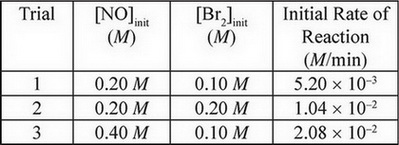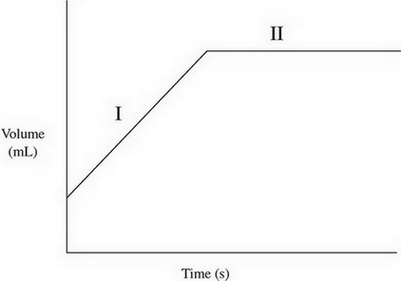416
Created byMaster Student

Chemistry Practice Test #2

1 / 30

14H+(aq) + Cr2O72-(aq) + 3Ni(s) →2Cr3+(aq) + 3Ni2+(aq) + 7H2O(l)

In the above reaction, a piece of solid nickel is added to a solution of potassium dichromate.

How many moles of electrons are transferred when 1 mole of potassium dichromate is mixed with 3 mol of nickel?

2 / 30

An unknown substance is found to have a high melting point. In addition, it is a poor conductor of electricity and does not dissolve in water. The substance most likely contains

3 / 30

Hydrogen fluoride, HF, is a liquid at 15°C. All other hydrogen halides (represented by HX, where X is any other halogen) are gases at the same temperature. Why?

4 / 30

2NO(g) + Br2(g) ↔ 2NOBr(g)

The above experiment was performed several times, and the following data was gathered:What is the rate law for this reaction?

5 / 30

Most transition metals share a common oxidation state of +2. Which of the following best explains why?

6 / 30

Neutral atoms of chlorine are bombarded by high-energy photons, causing the ejection of electrons from the various filled subshells. Electrons originally from which subshell would have the highest velocity after being ejected?

7 / 30

Which of the following pairs of ions would make the best buffer with a basic pH?

Ka for HC3H2O2 = 1.75 × 10-5

Ka for HPO42- = 4.8 × 10-13

8 / 30

2.The volume of a gas is charted over time, giving the above results. Which of the following options provides a possible explanation of what was happening to the gas during each phase of the graph?

9 / 30

Which of the following is true for all bases?

10 / 30

Which molecule or molecules exhibit sp2 hybridization around the central atom?

11 / 30

N2(g) + O2(g) + Cl2(g) ↔ 2NOCl(g) Δ = 132.6 kJ/mol

For the equilibrium above, what would happen to the value of Δ if the concentration of N2 were to increase and why?

12 / 30

A stock solution of 12.0 M sulfuric acid is made available. What is the best procedure to make up 100 mL of 4.0 M sulfuric acid using the stock solution and water prior to mixing?

13 / 30

Atoms of which element are most likely to form a structure with the formula XF6 (where X is one of the four atoms)?

14 / 30

14H+(aq) + Cr2O72-(aq) + 3Ni(s) → 2Cr3+(aq) + 3Ni2+(aq) + 7H2O(l)

In the above reaction, a piece of solid nickel is added to a solution of potassium dichromate.

Which species is being oxidized and which is being reduced? (Answer with the oxidized species first and the reduced species second)

15 / 30

4NH3(g) + 5O2(g) → 4NO(g) + 6H2O(g)

The above reaction will experience a rate increase by the addition of a catalyst such as platinum. Which of the following best explains why?

16 / 30

When in liquid state, which two substances are most likely to be miscible with water?

17 / 30

SF4(g) + H2O(1) → SO2(g) + 4HF(g) ΔH = -828 kJ/mol

Which of the following statements accurately describes the above reaction?

18 / 30

Which molecule would have the shortest bonds?

19 / 30

London dispersion forces are caused by

20 / 30

Between propane and ethene, which will likely have the higher boiling point and why?

21 / 30

A solution contains a mixture of four different compounds: KCl(aq), Fe(NO3)3(aq), MgSO4(aq), and N2H4(aq). Which of these compounds would be easiest to separate via distillation?

22 / 30

Which molecules are best represented by multiple resonance structures?

23 / 30

NH4+(aq) + NO2- (aq) → N2(g) + 2H2O(l)

Increasing the temperature of the above reaction will increase the rate of reaction. Which of the following is NOT a reason that increased temperature increases reaction rate?

24 / 30

Which of the following processes is an irreversible reaction?

25 / 30

A sample of oxygen gas at 50 °C is heated, reaching a final temperature of 100 °C. Which statement best describes the behaviour of the gas molecules?

26 / 30

14H+(aq) + Cr2O72-(aq) + 3Ni(s) → 2Cr3+(aq) + 3Ni2+(aq) + 7H2O(l)

In the above reaction, a piece of solid nickel is added to a solution of potassium dichromate.

How does the pH of the solution change as the reaction progresses?

27 / 30

The enthalpy value for a reaction is listed below

CH4(g) + H2(g) → C(s) + H2O(g) ΔH = -131 kJ/molrxn

How would the addition of a catalyst affect the enthalpy and entropy changes for this reaction? (Enthalpy change first, entropy change second)

28 / 30

A sample of an unknown chloride compound was dissolved in water and then titrated with excess Pb(NO3)2 to create a precipitate. After drying, it is determined there is 0.0050 mol of the precipitate present. What mass of chloride is present in the original sample?

29 / 30

 Initial pH pH after NaOH addition Acid 1 3.0 3.5 Acid 2 3.0 5.0

Two different acids with identical pH are placed in separate beakers. Identical portions of NaOH are added to each beaker, and the resulting pH is indicated in the table above. What can be determined about the strength of each acid?

30 / 30

A compound is made up of entirely silicon and oxygen atoms. If there are 14.00 g of silicon and 32.0 g of oxygen present, what is the empirical formula of the compound?